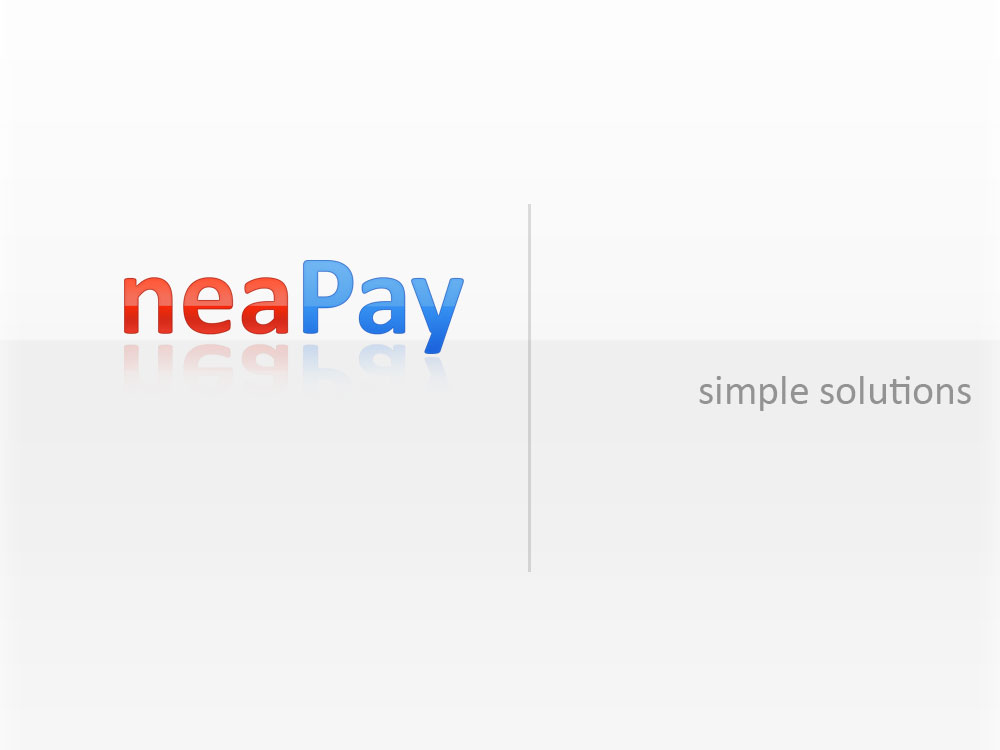Calculate Cryptogram ARQC/ARPC for EMV ISO8583

including key derivation and session key calculation

Just input the correct data from the ISO8583 Data Element 55, the EMV tags, then the keys and get the request and response cryptogram just like it was generated by the CHIP of the card

Calculate Cryptogram Request ARQC

9F02 Amount, Authorised (up to 12 digits, numeric, last 2 are decimals):

9F03 Amount, Other (up to 12 digits, numeric, last 2 are decimals, zero if none):

9F1A Terminal Country Code (4 hex digits, 2 bytes):

95 Terminal Verification Results TVR (10 hex digits, 5 bytes):

5F2A Transaction Currency Code (4 hex digits, 2 bytes):

9A Transaction Date (6 numeric digits):

9C Transaction Type (2 numeric digits):

9F37 Unpredictable Number UN (8 hex digits):

82 Application Interchange Profile (AIP) (4 hex digits):

9F36 Application Transaction Counter ATC (4 hex digits):

9F10 Issuer Application Data (IAD) (64 hex digits):

Cryptogram master key (32 hex digits):

Card Primary Account Number PAN (16-19 digits):

Card PAN Sequence number PSN:

Initialization Vector (32 hex digits, leave empty for zeroes):

Application Cryptogram (AC) (16 hex digits):

Calculate Cryptogram Response ARPC

Request Application Cryptogram (AC) (16 hex digits):

Card Status Update CSU (8 hex digits):

9F36 Application Transaction Counter ATC (4 hex digits):

Cryptogram master key (32 hex digits):

5A Card Primary Account Number PAN (16-19 digits):

Initialization Vector (32 hex digits):

How cryptogram calculation works

Terminal data is composed from the following fields:

Terminal Data: Amount, Authorised (Numeric)

Terminal Data: Amount, Other (Numeric)

Terminal Data: Terminal Country Code

Terminal Data: Terminal Verification Results

Terminal Data: Transaction Currency Code

Terminal Data: Transaction Date

Terminal Data: Transaction Type

Terminal Data: Unpredictable Number

ICC data is composed from the following fields:

ICC: Application Interchange Profile

ICC: Application Transaction Counter

Detailed cryptogram calculation data

DOL data:Are you ready to start or need help?

Ready to start your next project with us? Give us a call or send us an email and we will get back to you as soon as possible!

Get a free quote, Ask for details, Get guidance